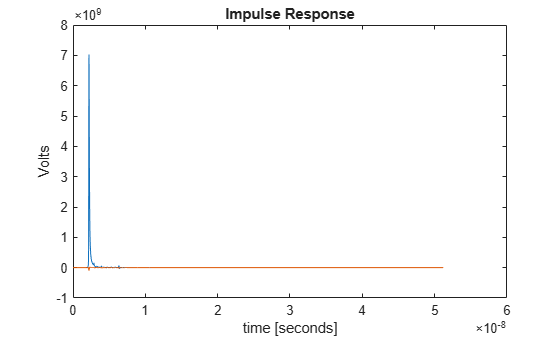# step2impulse

Impulse response from step response

## Syntax

``I = step2impulse(S,dt)``

## Description

example

````I = step2impulse(S,dt)` converts a step response `S` to an impulse response `I`, given the uniform sample interval `dt`.```

## Examples

collapse all

Load the step response column matrix from a file.

`load('StepResponseReflective100ps.mat');`

Calculate the impulse response from the step response.

`impulse = step2impulse(step,dt);`

Plot the step response.

```plot(t(1:end-1),impulse) xlabel('time [seconds]') ylabel('Volts') title('Impulse Response')```## Input Arguments

collapse all

Input step response, specified as a column matrix. The first column contains the primary step response and the subsequent columns (if any) contain the crosstalk step responses.

Data Types: `double`

Uniform timestep of the waveform, specified as a real positive scalar in seconds.

Data Types: `double`

## Output Arguments

collapse all

Converted impulse response, returned as a column matrix. The first column contains the primary impulse response and the subsequent columns (if any) contain the crosstalk impulse responses. The number of rows in the impulse response must be one less than the number of rows in the step response.

Data Types: `double`

## Extended Capabilities

### C/C++ Code GenerationGenerate C and C++ code using MATLAB® Coder™.